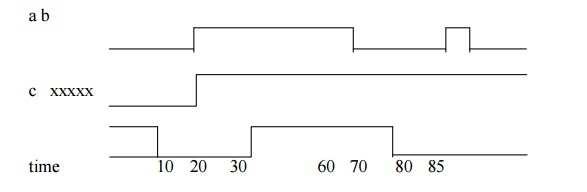Home | | VLSI Design | Data Flow and RTL

# Data Flow and RTL

In verilog coding gate-level modeling works well due to the number of gates are less; if large number of gates are used in a circuit then this type of modeling will be complicated.

DATA FLOW AND RTL

In verilog coding gate-level modeling works well due to the number of gates are less; if large number of gates are used in a circuit then this type of modeling will be complicated. Dataflow modeling is a powerful approach to implement large circuit. This modeling becomes a popular approach as logic synthesis tools have become difficult.

1. Continuous assignments:

Continuous assignments are one type of concurrent statements .  While gate

instantiations allow the description of a circuit’s structure, continuous assignments allow the description of a circuit’s function. General form of the continuous statement is assign net_assignment {, net_assignment};thenet_assignment can be any expression involving the operators.

Example:

assign carry = (a & b) | (a & c) | (b & c);

assign sum = a ^ b ^ c;

multiple assignments are specified in one assignment, using commas to separate the assignment.

Example:

assign carry = (a & b) | (a & c) | (b & c), sum = a ^ b ^ c;

Example for multibit assignment is wire [1:3] x, y, z;

assign z = x & y;

This results in z1 = x1y1, z2 = x2y2, z3 = x3y3. Delays in dataflow modeling:

Delay values control the time between the change in a right hand side operand and when thenew value is assigned to the left hand side. Delays in continuous assignment statements can be specified in three ways:

a)        Regular assignment delay

b)       Implicit continuous assignment delay

c)        Net declaration delay

2. Regular assignment delay:

In this way, the delay value can be specified after the keyword assign.

Example:

assign #10 c = a & b;1.        When the input a and b go to high at time 20, c goes to high at 10 time units later.

2.        When a goes low at 60, c goes to low at 70.

3.        When a changes to high at 80, but it goes down to low before 10 time units have over and done.

4.        So, at the time of recomputation, 10 units after 80, a is 0. Thus, c gets the value 0.

3. Implicit continuous assignment delay:

Implicit continuous assignment method is used to specify both a delay and an assignment on the net.

Example:

wire c;

assign #10 c = a & b;

In implicit continuous assignment delay we can write the above as:

wire #10 c = a & b;

4. Net declaration delay:

Net declaration delays can be used in gate level modeling. Delay can be specified on a net when it is declared without putting a continuous assignment on the net. If a delay is specified on a net c, then any value change applied to the net c is delayed accordingly.

Example:

wire c;

assign #10 c = a & b;

We can write the above by using net declaration delay as: wire #10 c;

assign c = a & b;

Study Material, Lecturing Notes, Assignment, Reference, Wiki description explanation, brief detail
VLSI Design : Specification Using Verilog HDL : Data Flow and RTL |